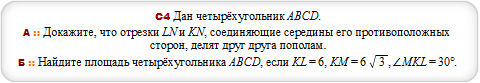# Закажи себе WolframAlpha|Pro! Получи пошаговые решения твоих задач!

## ЕГЭ с Wolfram|Alpha :: 19.05.14 :: Демон 2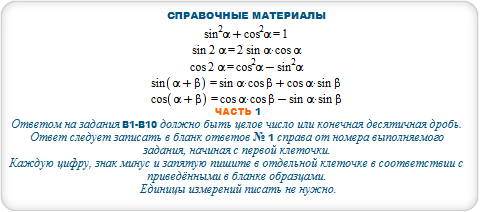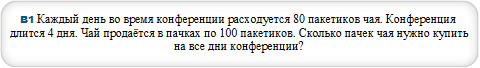#### ceil(a*b/c) for a= 80,b=4,c=100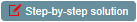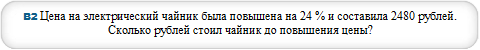#### s = n/(1+(1/100)*p) where n=2480,p=24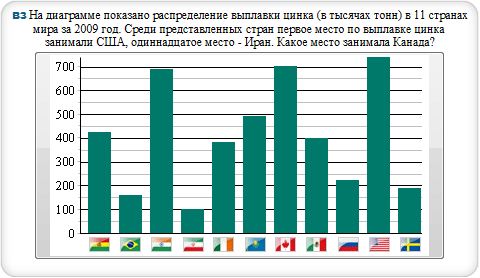#### BarChart(0,425,160,690,100,380,490,700,400,220,740,190)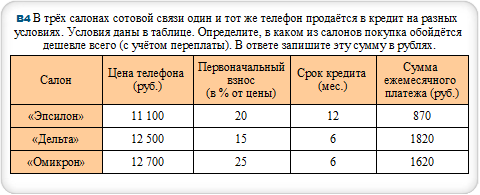#### X= (1/100)*a*A+k*u where a=20,A=11100,u=870,k=12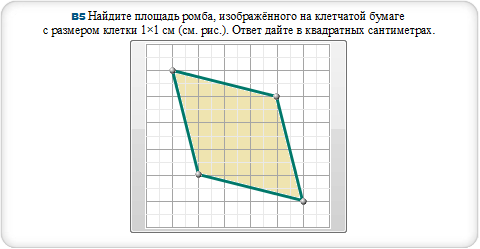#### polygon [0,5],[4,4],[5,0],[1,1]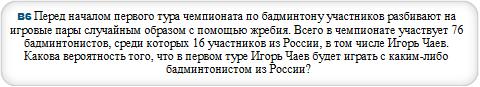#### p=(m-1)/(n-1) where m=16,n=76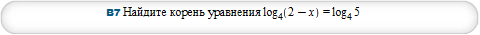#### log(4,2-x)=log(4,5)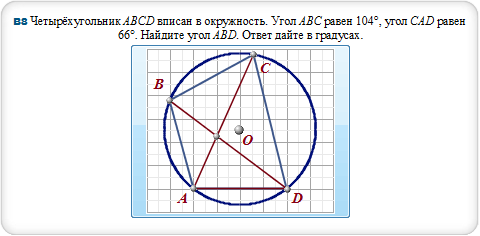#### polygon [-3,3.8],[2,0],[.6,5.8],[-2,0],[-3,3.8],[.6,5.8] and circle [-2,0],[-3,3.8],[.6,5.8]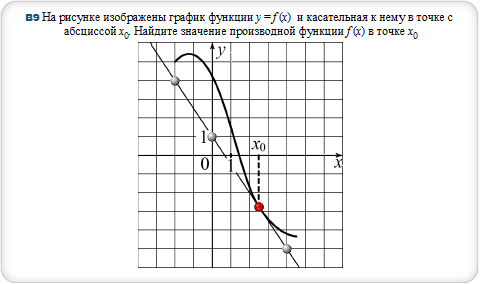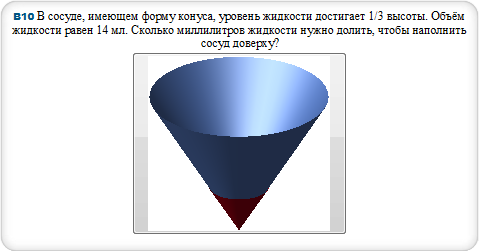#### cone with radius 3 and height 6, red cone with radius 1 and height 2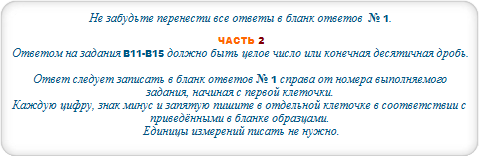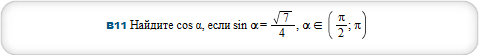#### cos(Pi-arcsin(sqrt7/4))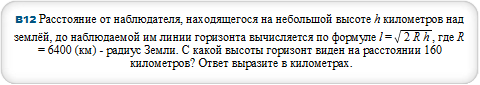#### N[l^2/(2*R)] where l=160,R=6400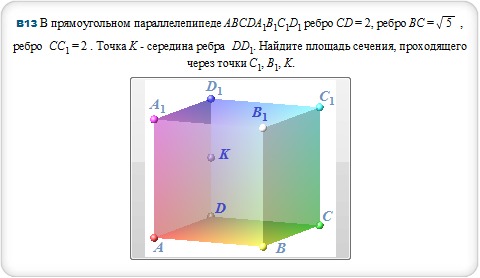#### [sqrt5,0,1],[sqrt5,0,2], [sqrt5,2,2],[0,0,1] and [sqrt5,0,0],[0,0,0],[0,2,0],[sqrt5,2,0]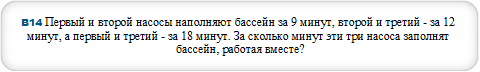#### t = 2/(1/k+1/m+1/n) for k=9,m=12,n=18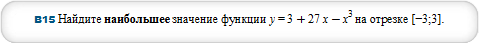#### maximize -x^3+27*x+3 over [-3,3]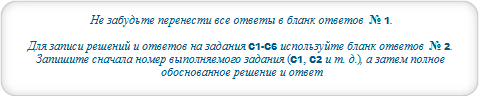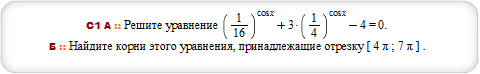#### (1/16)^cosx+3*(1/4)^cosx-4=0 over the reals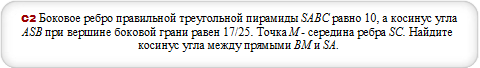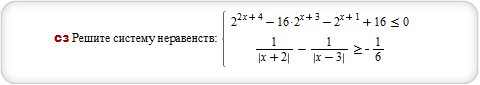#### solve {2^(2*x+4)-16*2^(x+3)-2^(x+1)+16<=0,1/abs(x+2)-1/abs(x-3) >=-1/6}